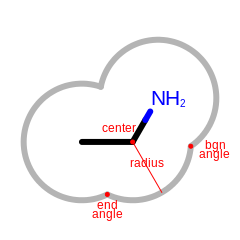OESurfaceArc¶

class OESurfaceArc

This class represents OESurfaceArc that stores data for drawing the arcs of molecule surface. See Figure: Example of the data stored in the OESurfaceArc class.Example of the data stored in the OESurfaceArc class

Note

Angles are interpreted such that $$0.0^{\circ}$$ and $$360.0^{\circ}$$ degrees are at the 12 o’clock position, $$90.0^{\circ}$$ degree corresponds to 3 o’clock, etc.

Constructors¶

OESurfaceArc()

Default constructor.

const OEDepict::OE2DPoint &center, double bgnAngle, double endAngle,

Creates an OESurfaceArc object with the given parameters.

OESurfaceArc(const OESurfaceArc &rhs)

Copy constructor.

operator=¶

OESurfaceArc &operator=(const OESurfaceArc &rhs)

Assignment operator.

GetAtomDisplay¶

const OEDepict::OE2DAtomDisplay *GetAtomDisplay() const

Returns the pointer of the OE2DAtomDisplay object the given arc belongs to.

GetBgnAngle¶

double GetBgnAngle() const

Returns the angle (in degrees) where the arc of the surface starts.

GetCenter¶

const OEDepict::OE2DPoint &GetCenter() const

Returns the (x,y) coordinates of the center of the surface arc.

GetEndAngle¶

double GetEndAngle() const

Returns the angle (in degrees) where the arc of the surface ends.

Returns the radius of the arc.

SetAtomDisplay¶

Sets the atom display to which the arc belongs.

SetBgnAngle¶

bool SetBgnAngle(double angle)

Sets the angle where the arc of the surface begins.

angle

The angle has to in the range of $$[0.0^{\circ}, 360.0^{\circ}]$$

SetCenter¶

bool SetCenter(const OEDepict::OE2DPoint &center)

Sets the (x,y) coordinates of the center of the surface arc.

SetEndAngle¶

bool SetEndAngle(double angle)

Sets the angle where the arc of the surface ends.

angle

The angle has to in the range of $$[0.0^{\circ}, 360.0^{\circ}]$$